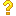•闪存
•博客
• 发言小组
• 投递新闻
• 提问博问
• 添加收藏
• 发布招聘
•文库

0

##分析代码，回溯法

#include<stdio.h>const MAX=11;int sum=0,temp=1; int k,n,i;int c[MAX];void s(int a, int b){ if(k==a)
4

##10c++ 返回值，临时变量。谁能帮忙分析以下代码。

(1) #include<iostream> using namespace std; class Test{ static int i; public: Test() { cout<<"ctor"<